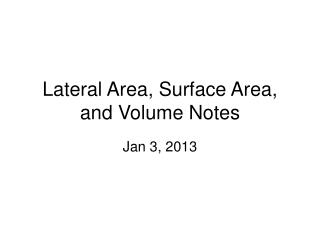# Lateral Area, Surface Area, and Volume Notes - PowerPoint PPT PresentationDownload PresentationLateral Area, Surface Area, and Volume Notes

Lateral Area, Surface Area, and Volume NotesDownload Presentation## Lateral Area, Surface Area, and Volume Notes

- - - - - - - - - - - - - - - - - - - - - - - - - - - E N D - - - - - - - - - - - - - - - - - - - - - - - - - - -
##### Presentation Transcript

1. Lateral Area, Surface Area, and Volume Notes Jan 3, 2013

2. Lateral Area Lateral area – these are the flat sides of prisms, cones, and cylinders lateral face There are 4 lateral faces on this shape and 2 bases

3. Definitions Lateral area – for prisms, cylinders and cones – the area of the figure not including the bases Surface Area – the sum of the area of all of the faces (sides and both bases) Volume – a measure of the amount of space contained in the interior of a solid

4. Prisms To find Lateral Area: perimeter times height or LA = ph 6 10 4

5. Lateral Area Identify the two bases. Find the perimeter of one base (add all the sides) Multiply the perimeter by the height 6 4 10 P = 4 + 4 + 6 + 6 = 20 20 (10) = 200

6. Lateral Area Perimeter of a 5 sided base is 5 x 10….p = 50 H = 12 50(12) = 600 Answer is 600cm2 10 cm 12cm Answers for area are always squared

7. Surface Area SA = LA + area of the 2 bases You need to remember the formulas for finding area and perimeter of solids! Steps: • Find p and h • Find LA • Find base area • Add 2 base areas and the LA to get SA • Final answer will be in units 2

8. Surface Area SA = LA + area of the 2 bases Find p of base (4 + 4 + 10 + 10) = 28 H = 17 17(28) = LA = 476 Area of rectangle  b x h Area of base = 4 x 10 = 40 SA = 40 + 40 + 476 = 556 SA = 556 cm 2

9. Surface Area SA = LA + area of the 2 bases The base is a parallelogram! P = 5 + 5 + 3 + 3 = 16 H = 2 2(16) = 32 = LA A = b (3) h (5) A = 15 SA = 32 + 15 + 15 = 62m2 5m 3m 2m

10. Volume Volume is the space contained within a shape. To find volume, find the area of the base and multiply it with the height ***Remember the perimeter of a circle is circumference (2πr) and area is πr2

11. Volume Volume = b x h Area of a triangle is ½ (b x h) ½ (3 x 2 ) = 3 3 x 4 = 12 Answer = 12cm3 2cm 4cm 3cm